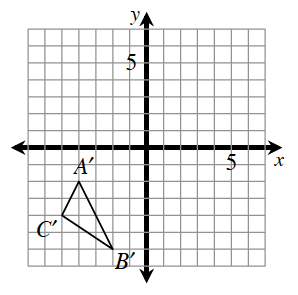### Home > GC > Chapter 5 > Lesson 5.3.5 > Problem5-118

5-118.$ΔABC$ was reflected across the $x\text{-axis}$, and then that result was rotated $90º$ clockwise about the origin to result in $ΔA'B'C'$, shown at right. Find the coordinates of points $A$, $B$, and $C$ of the original triangle.

To undo the transformation, rotate $ΔA'B'C'$ $90º$ counter-clockwise then reflect the result across the $x\text{-axis}$.

$A(2,4)$$B(6,2)$$C(4,5)$

Use the eTool below to explore the process of undoing the transformation.
Click the link at right for the full version of the eTool: 5-118 HW eTool